# Converting Percents and Decimals

Recall that a percent shows a part out of 100. By knowing that we are looking for part of 100 makes converting much simpler.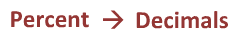Example #1: 23%

23% means 23 out of 100. We can write this as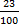This is read as 23 hundredths, which is written as23% = 0.23

Example #2: 48%

Let's try another. 48% =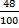= 0.48

Example #3: 9%

9% == 0.09

This can be tougher if the percent is less than 1% or greater than 100%.

Example #4: 0.5%

0.5% =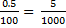= 0.005

Example #5: 230%

230% == 2.3We can use place value to help us convert from decimals to percents as well.

Example #6: 0.19

0.19 is read as 19 hundredths. So we can write this as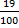.

Now that we have this written as a part out of 100, we have a percent.

0.19 = 19%

Example #7: 0.25

0.25 =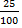= 25%

(In examples like this one, be sure not to reduce the fraction.
You want the denominator to be 100 to convert to a percent.)

Example #8: 0.4

0.4 =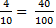= 40%

Example #9: 1.3

1.3 = 1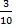= 1130%

Notice that when the decimal is over 1, the percent is over 100%.

Example #10: 0.003

0.003 =0.3%

In this example, the decimal is less than 1 one-hundredth.
Therefore, the percent is less than 1.

A Short Cut Now that you have seen why it works, here is the short cut:

To go from percent to decimal, move the decimal two times to the left.

45% = 045 = 0.45

To go from decimal to percent, move the decimal two times to the right.

0.67 = 067 = 67%